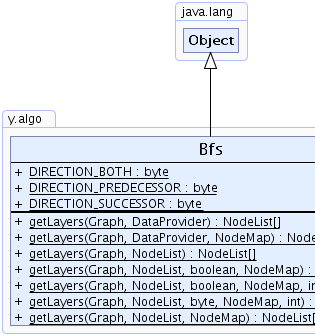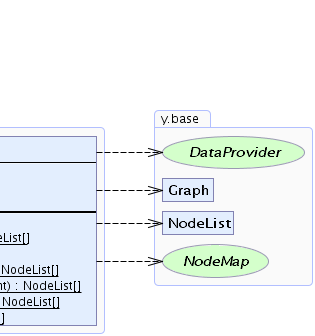Search this API

## y.algo Class Bfs

```java.lang.Objecty.algo.Bfs
```

`public class Bfsextends java.lang.Object`

This class provides services that center around breadth first search (BFS).

Breadth first search starts at a given set of nodes and explores the neighboring nodes first, before visiting the next level neighbors.

A breadth first search run can be either directed or undirected. All methods require a list of nodes that are considered as the core nodes from which the breadth first search starts. These nodes can be identified either with a `NodeList` or with a `DataProvider` that returns `true` for core nodes and `false` for all other nodes. The output is given as an array of `NodeList`s each of which contains the nodes of a particular layer.Example for a BFS run. The marked node is the core node from which BFS starts while node labels indicate the resulting layers.Field Summary
`static byte` `DIRECTION_BOTH`
An edge direction specifier for both incoming and outgoing edges.
`static byte` `DIRECTION_PREDECESSOR`
An edge direction specifier for incoming edges.
`static byte` `DIRECTION_SUCCESSOR`
An edge direction specifier for outgoing edges.

Method Summary
`static NodeList[]` ```getLayers(Graph graph, DataProvider isCoreNode)```
Returns the layers of nodes constructed by a breadth first search.
`static NodeList[]` ```getLayers(Graph graph, DataProvider isCoreNode, NodeMap layerIDMap)```
Returns the layers of nodes constructed by a breadth first search.
`static NodeList[]` ```getLayers(Graph graph, NodeList coreNodes)```
Returns the layers of nodes calculated by a breadth first search.
`static NodeList[]` ```getLayers(Graph graph, NodeList coreNodes, boolean directed, NodeMap layerIDMap)```
Returns the layers of nodes constructed by either a directed or undirected breadth first search.
`static NodeList[]` ```getLayers(Graph graph, NodeList coreNodes, boolean directed, NodeMap layerIDMap, int maxLayers)```
Returns the layers of nodes constructed by a directed/undirected breadth first search where the maximum number of layers is restricted.
`static NodeList[]` ```getLayers(Graph graph, NodeList coreNodes, byte direction, NodeMap layerIDMap, int maxLayerCount)```
Returns the layers of nodes constructed by a directed/undirected breadth first search where the maximum number of layers is restricted.
`static NodeList[]` ```getLayers(Graph graph, NodeList coreNodes, NodeMap layerIDMap)```
Returns the layers of nodes constructed by a breadth first search.

Methods inherited from class java.lang.Object
`clone, equals, finalize, getClass, hashCode, notify, notifyAll, toString, wait, wait, wait`

Field Detail

### DIRECTION_PREDECESSOR

`public static final byte DIRECTION_PREDECESSOR`
An edge direction specifier for incoming edges.

`getLayers(y.base.Graph, y.base.NodeList, byte, y.base.NodeMap, int)`, Constant Field Values

### DIRECTION_SUCCESSOR

`public static final byte DIRECTION_SUCCESSOR`
An edge direction specifier for outgoing edges.

`getLayers(y.base.Graph, y.base.NodeList, byte, y.base.NodeMap, int)`, Constant Field Values

### DIRECTION_BOTH

`public static final byte DIRECTION_BOTH`
An edge direction specifier for both incoming and outgoing edges.

`getLayers(y.base.Graph, y.base.NodeList, byte, y.base.NodeMap, int)`, Constant Field Values
Method Detail

### getLayers

```public static NodeList[] getLayers(Graph graph,
NodeList coreNodes)```
Returns the layers of nodes calculated by a breadth first search.

The first of these layers contains all nodes within the given `NodeList`. These nodes are the core nodes from which an undirected breadth first search to the other nodes starts.

In the `i`-th layer are previously unassigned nodes that are connected to nodes in the `(i-1)`-th layer.

Complexity:
`O(graph.N() + graph.E())`
Parameters:
`graph` - the given graph
`coreNodes` - the list of core nodes from which the BFS starts
Returns:
an array of `NodeList`s each of which contains the nodes of a particular layer

### getLayers

```public static NodeList[] getLayers(Graph graph,
DataProvider isCoreNode)```
Returns the layers of nodes constructed by a breadth first search.

The first of these layers contains all nodes for which the given `DataProvider` returns `true`. These nodes are the core nodes from which an undirected breadth first search to the other nodes starts.

In the `i`-th layer are previously unassigned nodes that are connected to nodes in the `(i-1)`-th layer.

Precondition:
`isCoreNode.getBool(node)` defined for all nodes in graph
Parameters:
`graph` - the given graph
`isCoreNode` - the `DataProvider` that contains the nodes from which the BFS starts; core nodes are marked with a `true` value
Returns:
an array of `NodeList`s each of which contains the nodes of a particular layer

### getLayers

```public static NodeList[] getLayers(Graph graph,
DataProvider isCoreNode,
NodeMap layerIDMap)```
Returns the layers of nodes constructed by a breadth first search.

The first of these layers contains all nodes for which the given `DataProvider` returns `true`. These nodes are the core nodes from which an undirected breadth first search to the other nodes starts. The algorithm fills the provided `NodeMap` with an integer indicating the layer of each node.

In the `i`-th layer are previously unassigned nodes that are connected to nodes in the `(i-1)`-th layer.

Precondition:
`isCoreNode.getBool(node)` defined for all nodes in graph
Parameters:
`graph` - the given graph
`isCoreNode` - the `DataProvider` that contains the nodes from which the BFS starts; core nodes are marked with a `true` value
`layerIDMap` - the `NodeMap` that will be filled during the BFS execution and holds the zero-based index of the BFS layer to which each node belongs or `-1` if the node is not reachable
Returns:
an array of `NodeList`s each of which contains the nodes of a particular layer

### getLayers

```public static NodeList[] getLayers(Graph graph,
NodeList coreNodes,
NodeMap layerIDMap)```
Returns the layers of nodes constructed by a breadth first search.

The first of these layers contains all nodes within the given `NodeList`. These nodes are the core nodes from which an undirected breadth first search to the other nodes starts. The algorithm fills the provided `NodeMap` with an integer indicating the layer of each node.

In the `i`-th layer are previously unassigned nodes that are connected to nodes in the `(i-1)`-th layer.

Precondition:
`isCoreNode.getBool(node)` defined for all nodes in graph
Parameters:
`graph` - the given graph
`coreNodes` - the list of core nodes from which the BFS starts
`layerIDMap` - the `NodeMap` that will be filled during the BFS execution and holds the zero-based index of the BFS layer to which each node belongs or `-1` if the node is not reachable
Returns:
an array of `NodeList`s each of which contains the nodes of a particular layer

### getLayers

```public static NodeList[] getLayers(Graph graph,
NodeList coreNodes,
boolean directed,
NodeMap layerIDMap)```
Returns the layers of nodes constructed by either a directed or undirected breadth first search.

The first of these layers contains all nodes within the given `NodeList`. These nodes are the core nodes from which a directed/undirected breadth first search to the other nodes starts. The algorithm fills the provided `NodeMap` with an integer indicating the layer of each node.

In the `i`-th layer are previously unassigned nodes that are connected to nodes in the `(i-1)`-th layer.

Complexity:
`O(graph.N() + graph.E())`
Parameters:
`graph` - the given graph
`coreNodes` - the list of core nodes from which the BFS starts
`directed` - `true` if the graph should be considered directed, `false` otherwise
`layerIDMap` - the `NodeMap` that will be filled during the BFS execution and holds the zero-based index of the BFS layer to which each node belongs or `-1` if the node is not reachable
Returns:
an array of `NodeList`s each of which contains the nodes of a particular layer

### getLayers

```public static NodeList[] getLayers(Graph graph,
NodeList coreNodes,
boolean directed,
NodeMap layerIDMap,
int maxLayers)```
Returns the layers of nodes constructed by a directed/undirected breadth first search where the maximum number of layers is restricted.

The first of these layers contains all nodes within the given `NodeList`. These nodes are the core nodes from which either a directed or undirected breadth first search to the other nodes starts. The algorithm fills the provided `NodeMap` with an integer indicating the layer of each node.

In the `i`-th layer are previously unassigned nodes that are connected to nodes in the `(i-1)`-th layer.

If the number of layers is restricted, the returned `NodeList` may only contain a subset of nodes (i.e., only those with layer <= max layer).
Complexity:
`O(graph.N() + graph.E())`
Parameters:
`graph` - the given graph
`coreNodes` - the list of core nodes from which the BFS starts
`directed` - `true` if the graph should be considered directed, `false` otherwise
`layerIDMap` - the `NodeMap` that will be filled during the BFS execution and holds the zero-based index of the BFS layer to which each node belongs or `-1` if the node is not reachable
`maxLayers` - the number of layers that will be returned or `0` if all layers are required
Returns:
an array of `NodeList`s each of which contains the nodes of a particular layer

### getLayers

```public static NodeList[] getLayers(Graph graph,
NodeList coreNodes,
byte direction,
NodeMap layerIDMap,
int maxLayerCount)```
Returns the layers of nodes constructed by a directed/undirected breadth first search where the maximum number of layers is restricted.

The first of these layers contains all nodes within the given `NodeList`. These nodes are the core nodes from which a breadth first search to the other nodes starts. The algorithm fills the provided `NodeMap` with an integer indicating the layer index of each node (all core nodes have layer index `0`).

In the `i`-th layer are previously unassigned nodes that are connected to nodes in the `(i-1)`-th layer.

If the number of layers is restricted, the returned `NodeList` may only contain a subset of nodes (i.e., only those with layer index < max layer count).
Complexity:
`O(graph.N() + graph.E())`
Parameters:
`graph` - the given graph
`coreNodes` - the list of core nodes
`direction` - one of the predefined direction specifiers
`layerIDMap` - the `NodeMap` that will be filled during the BFS execution and holds the zero-based index of the BFS layer to which each node belongs or `-1` if the node is not reachable
`maxLayerCount` - the maximum number of layers that will be returned or `0` if all layers are required
Returns:
an array of `NodeList`s each of which contains the nodes of a particular layer
Throws:
`java.lang.IllegalArgumentException` - if the given direction is not supported